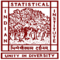Poisson Distribution: Definition and Examples

# Poisson Distribution: Definition and Examples

clickHere
Updated on Dec 9, 2022 08:46 IST

## Introduction:

A probability distribution is a function that gives the relationship between the outcome of a random variable in any random experiment and its probable values. In this article, we will discuss one of the probability distributions which is commonly used in Data Science, Poisson Distribution : Definition and Example.

To know more about Random Variable, read the article Introduction to Probability.

To know about other probability distributions, read the article Probability Distribution used in Data Science.

## Poisson Distribution:

Poisson Distribution (named after the French mathematician Denis Simon Poisson) is a discrete probability distribution that measures the probability of a random variable over a specific period of time.

Example:

• Number of arrivals at a restaurant
• Number of calls per hour in a call center

## Conditions for Poisson Distribution:

• An event can occur any number of times in the defined period of time
• All the events are independent
• The rate of occurrence of events is constant

## Mathematical Definition:

For any random variable X, the distribution function for Poisson Distribution is given by:

To know more about the mean, read the article on Measures of Central Tendency.

Example: Poisson distribution using Python

1.

```# import libraries

import matplotlib.pyplot as plt
from scipy.stats import poisson #poisson: poisson distribution function

# generating poisson distribution for sample size of 1000

sample_set = poisson.rvs(mu = 5, size = 1000) #poisson.rvs : generate the random number

# plot the poisson distribution

plt.hist(sample_set, edgecolor = 'red')```

2. Poisson distribution at the different mean values for the same sample size

```# import library

from numpy import random
import matplotlib.pyplot as plt
import seaborn as sns

# plotting poisson distribution for different mean values
# lam : mean value
sns.distplot(random.poisson(lam=20, size=1000), hist=False, label='mean = 20')
sns.distplot(random.poisson(lam=50, size=1000), hist=False, label='mean = 50')
sns.distplot(random.poisson(lam=80, size=1000), hist=False, label='mean = 80')

plt.legend()
plt.show()```

From the above figure, we get as the mean value increase the curve become flatter and shorter.

## Properties of Poisson Distribution:

• Poisson distribution has only one parameter i.e. mean
• Mean = Variance
• It tends to normal distributions, if mean tends to infinity

To know more about normal distribution, read the article on Normal Distribution: Definition and Example.

## Relation between Poisson and Binomial Distribution:

Poisson distribution is a limiting case of Binomial Distribution.

i.e. when we increase the value of n to infinite we get the Poisson distribution.

The distribution function of Binomial Distribution is given by:

To know more about Binomial distribution, read the article Binomial Distribution: Definition and Example.

Now, substituting the value of p in B(x: n, p), we get:

This is the required Poisson distribution function.

## Conclusion:

In this article, we have discussed about one of the most important probability distribution Poisson Distribution , with examples in python.

Top Trending Articles:
Data Analyst Interview Questions | Data Science Interview Questions | Machine Learning Applications | Big Data vs Machine Learning | Data Scientist vs Data Analyst | How to Become a Data Analyst | Data Science vs. Big Data vs. Data Analytics | What is Data Science | What is a Data Scientist | What is Data Analyst

clickHere

Vikram has a Postgraduate degree in Applied Mathematics, with a keen interest in Data Science and Machine Learning. He has experience of 2+ years in content creation in Mathematics, Statistics, Data Science, and Mac... Read Full Bio

## Trending Data Science CoursesMicroMasters® Program in Statistics and Data ScienceMicroMasters® Program in Statistics and Data Scien...
edX3.7## Top Picks & New Arrivals